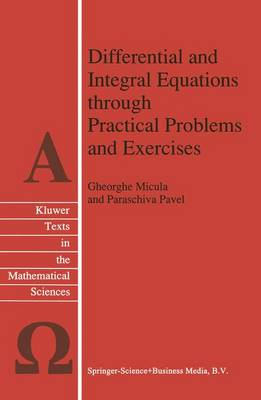•Differential and Integral Equations through Practical Problems and Exercises - Texts in the Mathematical Sciences 7 (Paperback)

(author), (author)
£119.99
Paperback 397 Pages / Published: 15/12/2010
• We can order this

Usually dispatched within 3 weeks

Many important phenomena are described and modeled by means of differential and integral equations. To understand these phenomena necessarily implies being able to solve the differential and integral equations that model them. Such equations, and the development of techniques for solving them, have always held a privileged place in the mathematical sciences. Today, theoretical advances have led to more abstract and comprehensive theories which are increasingly more complex in their mathematical concepts. Theoretical investigations along these lines have led to even more abstract and comprehensive theories, and to increasingly complex mathematical concepts. Long-standing teaching practice has, however, shown that the theory of differential and integral equations cannot be studied thoroughly and understood by mere contemplation. This can only be achieved by acquiring the necessary techniques; and the best way to achieve this is by working through as many different exercises as possible. The eight chapters of this book contain a large number of problems and exercises, selected on the basis of long experience in teaching students, which together with the author's original problems cover the whole range of current methods employed in solving the integral, differential equations, and the partial differential equations of order one, without, however, renouncing the classical problems. Every chapter of this book begins with the succinct theoretical exposition of the minimum of knowledge required to solve the problems and exercises therein.

Publisher: Springer
ISBN: 9789048141845
Number of pages: 397
Weight: 623 g
Dimensions: 240 x 160 x 21 mm
Edition: Softcover reprint of hardcover 1st ed. 1992

MEDIA REVIEWS
`This excellent and invaluable problem book can be recommended to teachers, students (both graduate and undergraduate) and also interested in differential and integral equations.'
Acta Science Mathematics, 59 (1994)